The Evaluation Process

When evaluating an expression the mathematica kernel applies a collection  of rules to an expression until the expression no longer changes.  In the  discussion below I explain the evaluation process in greater depth.  This  discussion of the evaluation process is based on a tutorial by David Withoff  which is posted at   http://library.wolfram.com/infocenter/Conferences/4683/.   That tutorial applied to Mathematica 2.0, and further details to reflect changes in Mathematica 3.0 come from  Power Programming With Mathematica the kernel (by David Wagner).  The book by David Wagner is apparently out  of print.  As far as I know there were no changes to the evaluation process  in Mathematica 4 or Mathematica 5.

This discussion doesn't cover the use of MakeExpression, \$PreRead,  \$Pre, \$Post, \$PrePrint, FormatValues, and MakeBoxes.  That is all discussed  in an earlier section.  Each step discussed there requires completing the evaluation process  discussed in this section.

Furthermore, this discussion doesn't address  the pattern matching process.

Steps of Evaluating of  h[a1, a2, a3]

Below I talk about "external DownValues", "internal DownValues" and  likewise for UpValues, SubValues, and NValues.  External values are those  that are not part of the Mathematica kernel while internal values are those that are part of the kernel.

The 17 steps below are repeated until an expression no longer changes, and  the decision to end the process happens in step 3 below.  When the expression  being evaluated does change the whole process starts again at the begininig  on the new expression.  In pathalogical cases such as in the next cell  evaluation completes due to exceeding \$IterationLimit.  In other cases  evaluation completes due to exceeding \$RecursionLimit.  I don't discuss how  counting iterarions and recursions fit into the evaluation process because I  have never seen an explanation of when that happens.This discussion overlooks the fact that NHoldAll, NHoldRest and NHoldFirst
prevent N from approximating one or more argument of affected expressions.

Also the functions Plus and Times use internal UpValues and internal
DownValues before external definitions, but that detail is overlooked
below.

Step 1
If the expression being evaluated is a symbol with an OwnValue replace  it with the OwnValue.  OwnValues are used when some value is assigned to a symbol (e.g.   x=5).

Step 2
Evaluate the head of the expression.

Step 3
If no part of the expression has changed during the last time around  this procedure, return the expression.  This is an optimazation that prevents  unecessary reevaluation of large expressions.

Step 4
If  (h) has the HoldAllComplete attribute skip to step 11 below. HoldAllComplete prevents any changes to  the arguments of a function.

If (h) doens't have attributes that prevent  evaluation of some or all arguments, the argumenrts evaluate from left to  right.  When (h) has either attribute (HoldFirst, HoldRest, HoldAll) evaluation continues without evaluating the affected arguments.  If any  of the arguments have the head Unevaluated, then the head Unevaluated is removed and further evaluation of the  argument is prevented.  In step 17 the head Unevaluated may be restored to  the argument.

If (h) has either attribute (HoldFirst, HoldRest, HoldAll),  and an argument of (h) has the head Evaluate, then the argument evaluates  even if the attribute would have prevented evaluation.

Step 5
If (h) has the Flat attribute flatten layers of (h) as in the next cell.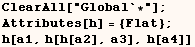Step 6
If (h) does not have the SequenceHold attribute splice together sequences.
In the next cell Sequences are  spliced together.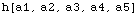Next (h) has the SequenceHold attribute and sequences aren't spliced.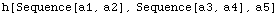Step 7
If (h) has the Listable attribute thread (h) over Lists.
This has the  same effect as evaluating  Thread[ h[a1,a2,a3] ]  when (h) isn't Listable.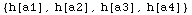Step 8
If (h) has the Orderless attribute sort the arguments of (h).
Note  the arguments are sorted in canonical order which may not be the same as  numeric order when the arguments are numeric.  This is seen in the next  cell.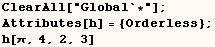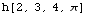Step 9
Use external Upvalues for the symbolic head of of each argument of the expression.  For example  in the next cell we would use the external UpValues of (g1), then the  external UpValues of (g2),  then the external UpValues of Derivative are  used.

The "symbolic head" of an expression is the result of nesting Head  until a symbol is returned.    Notice Derivative is the symbolic head of.  Here we have to nest head three times to get a symbol.  The head of ofis (f ' ), and the head of that is Derivative, which finally has the  head Derivative.Step 10
Use internal UpValues for the symbolic head of of each argument of the  expression.  For example in the previous cell we would use the internal  UpValues of (g1), then the internal UpValues of (g2),  then the internal  UpValues of Derivative are used.

Step 11
If (h) inis a symbol, the external DownValues for (h) are used.

Step 12
If (h)  in h[g1[a], g2[a], g3[a]]  is not a symbol the external SubValues of (h) are used.  The head of the expression in the next cell is h.   Since (h) isn't a symbol the Subvalues of (h) would be used.Step 13
If (h) in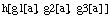is a symbol, the internal DownValues for (h) are used.

Step 14
If (h)  in h[g1[a], g2[a], g3[a]]  is not a symbol the internal  SubValues of (h) are used.

Step 15
Use external NValues if the expression being evaluated has the head N.   More precisely the NValues of the symbolic head of the first argument of N are used.

Step 16
Use internal NValues if the expression being evaluated has the head N.   As in the previous step the NValues of the symbolic head of the first  argument of N are used.

Step 17
If no UpValues, DownValues, SubValues, or NValues were used, and any  arguments of (h) did have the head Unevaluated. Then the head Unevaluated is  restored to that argument.  This is demonstrated in the next cell.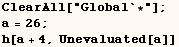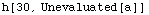Unevaluated prevents sequences from splicing, and prevents attributes such as
Flat and Orderless from taking effect.  This is demonstrated in the next
cell.However, in the next cell (h) has a downvalue that applies and we don't see
Unevaluated in the result.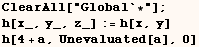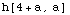Some Examples

From the discussion above it may be hard to understand the order that parts
of a complicated expression evaluate.  I provide two examples below to
demonstrate.  First evaluate the next cell which makes some definitions we
will use.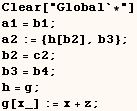Consider evaluation of the example in the next cell.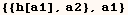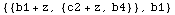You could see the order of evaluation using Trace or a similar function, but
I find the output of these functions difficult to read. Instead I like to use
Print statements as I do below.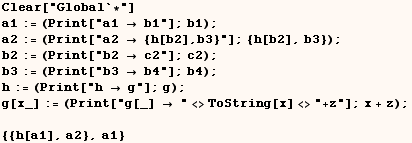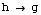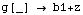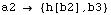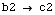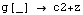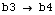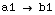Next consider how expressions evaluate when the head (h) in h[x] is a
non-trivial expression.  The next cell gives one such example.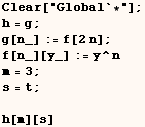The next cell shows how evaluation in this example progresses.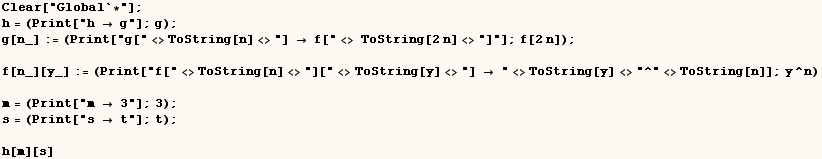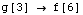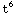Created by Mathematica  (May 17, 2004)